https://doi.org/10.5194/ms-14-15-2023
https://doi.org/10.5194/ms-14-15-2023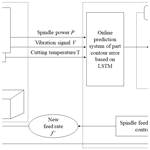# Short communication: Part contour error prediction based on LSTM neural network

Yun Zhang, Guangshun Liang, Cong Cao, Yun Zhang, and Yan Li
Abstract

Machine tools are subject to multiple sources of error during machining, resulting in deviations in the dimensions of the part and a reduction in contour accuracy. This paper proposes a contour error prediction model based on a long short-term memory (LSTM) neural network, taking hexagonal recess machining as an example and considering the power, vibration, and temperature signals that affect the contour error. The experimental data show that the model can accurately predict the contour error of the machined part. A more accurate and robust contour error prediction model can provide data support for online compensation of contour errors.

Share
Dates
1 Introduction

A computer numerical control (CNC) machine tool is the most influential piece of equipment for the national manufacturing industry. According to relevant studies, among the various types of errors in CNC machine tools, geometric errors and thermal errors account for 40–70 % of the total errors in CNC machine tool machining systems (Liu et al., 2017; Lu et al., 2020; Miao et al., 2015; Niu at al., 2021; Zhou et al., 2017; Zhu et al., 2012). In order to reduce the impact of machine tool errors on the machining accuracy of parts, the contour errors of parts are predicted and compensated in advance.

Scholars at home and abroad have been working a lot on the problem of machining accuracy prediction and compensation. Xiong et al. (2018) established a BP (back propagation) neural network prediction evaluation model for machining error by taking 12 parameters, such as machine structure and accuracy parameters, part feature parameters, and machining process parameters, as inputs to the machining error prediction model. The results show that the data obtained by the BP neural network model training are basically consistent with the coordinate measuring machine (CMM) measurement data of the sample parts, and the selected evaluation indexes are valid. Abdulshahed et al. (2015) proposed a thermal error modeling method based on adaptive neuro-fuzzy inference system (ANFIS) in order to build the relationship between thermal error and temperature variation. The results show that the ANFIS model by using the fuzzy C-means clustering method (ANFIS-FCM) has high prediction accuracy and fewer rules. The residual values of the model are less than ± 4 µm.

Long short-term memory (LSTM) has been widely applied to error modeling and prediction of machine tools. To address the problem of ignoring the effects of electronic control data and time series in the modeling of feed axis thermal error, Huang et al. (2021) proposed a thermal error prediction model based on LSTM neural networks that considered temperature variations and electronic control data. The experimental results show that the prediction accuracy and robustness of the model can be improved by considering temperature variation and electronic control data when modeling the thermal error of the feed axis of CNC machine tools.

Finding the integrated relationship between spindle power, vibration signal, cutting temperature, and error requires a lot of work, and the data processing is complicated. To address this problem, this paper establishes a contour error prediction model based on LSTM neural network similar to the black box model. The prediction model is trained by actually measuring the CNC machine tool spindle power, vibration signal, cutting temperature, and the contour error of the part, and the prediction effect of the model is evaluated. The results of the study provide an important foundation for adaptive control of the CNC machine tool spindle feed rate, which balances machine accuracy and efficiency. It is shown in Fig. 1.

2 Model training and result analysis

## 2.1 Acquisition of training samples

The processing object of this paper is a hexagonal recess, and the processing material is aluminum alloy 6061.The length and width of the workpiece are 85 mm and the height is 18 mm. The vertical machining center (VMCL1100) is selected for machining experiments, and the tool material is carbide. The diameter of the tool is 6 mm and the number of teeth is 2. Rough machining is conducted first, leaving a margin of 0.1 mm, and then finish machining is processed. For finishing, the spindle speed is 6500 r min−1 and the feed rate is 1000 mm min−1. Due to the arrangement of multiple sensors, there is no cutting lubricant in the milling process.

The three-phase AC power analyzer AWS2103 was used to measure the active power of the machine tool spindle with the finishing process as the study object. The vibration signal during the machining process is obtained with a three-way acceleration sensor ULT2017. The K-type thermocouple and temperature patrol UT3208 were used to obtain the temperature changes of some heat-generating parts of the CNC machine tool.

Table 1Comparison of the prediction effect of LSTM with bidirectional long short-term memory (BiLSTM) and gate recurrent unit (GRU).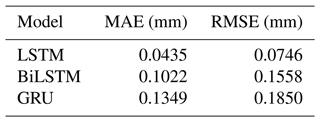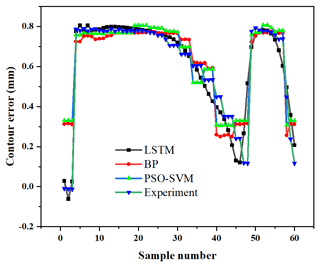Figure 2Comparison of predicted values of LSTM, BP, and PSO-SVM (particle swarm optimized support vector machine).

Table 2Comparison of the prediction effect of LSTM and traditional methods.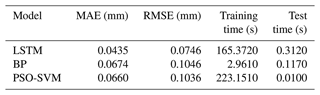## 2.2 Prediction effect of LSTM model

A total of 360 sets of power, vibration acceleration, temperature, and contour error data are collected as sample time series data. Among them, the first 300 sets of data are training samples and the remaining 60 sets of data are test samples.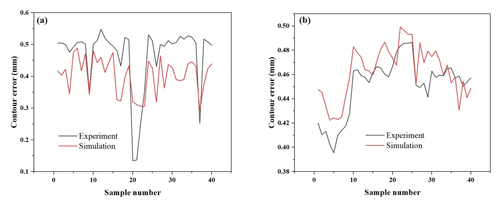Figure 3Prediction effect of contour error in (a) experiment 1 and (b) experiment 2.

To verify the effectiveness of the contour error prediction model based on LSTM neural network, the same test samples were used, the same network parameters were set, and then BiLSTM and GRU neural networks were built. The complete prediction models were obtained by training, and the prediction effects of the three models, LSTM, BiLSTM, and GRU, were subsequently verified using the test samples.

These three models all contain three layers of LSTM layer. And the number of units in each layer is 64, 128, and 256, respectively. The maximum number of iterations is 250, and the initial learning rate is 0.005. After 125 times of training, the learning rate decreases, and the drop factor is 0.2.

Mean absolute deviation (MAE) and root mean square error (RMSE) are used to evaluate the prediction performance of algorithms. Both can reveal the performance of the algorithm from different perspectives. The prediction effects are evaluated as shown in Table 1. The MAE and RMSE of the LSTM model are smaller than those of the BiLSTM model and the GRU model. Therefore, the part contour error prediction model based on LSTM recurrent neural network proposed in this paper obtains a better prediction effect.

## 2.3 Comparative analysis with traditional models

The conventional back propagation (BP) neural network prediction model and particle swarm optimized support vector machine (PSO-SVM) prediction model were constructed using the aforementioned data samples. The first 300 groups of data are training samples, and the remaining 60 groups of data are test samples. The number of hidden layer units of BP algorithm is 5, the number of training times is 250, the learning rate is 0.005, and the minimum error of training target is 0.001. The evolution time of PSO-SVM model is 250, the population size is 30, and the two parameters of the model are c1= 1.6 and c2= 1.5. The prediction values of BP and PSO-SVM are shown in Fig. 2.

MAE, RMSE, training time, and testing time are shown in Table 2. It can be seen that the MAE and RMSE of the LSTM model are smaller than those of the PSO-SVM model and the BP model, so the LSTM has higher prediction accuracy than the traditional method.

In this paper, the BP model has the shortest training time and the PSO-SVM model has the shortest testing time. In general, the training is performed on the collected data when the machine tool is offline. And during normal operation of the machine, the real-time data collected by the sensors are fed into the trained model to predict the current contour error value. This predicted value can be used for adaptive adjustment of speed and error compensation to improve machining accuracy. Therefore, test time is more important than training time, and shorter test time means faster output of predicted values. The appropriate algorithm is selected based on the actual model accuracy and run-time requirements.

3 Robustness analysis of LSTM model

On the CY-VMC1160L vertical machining center, hexagonal grooves are machined at different feed speeds to study the robustness of LSTM model. The experiment with a feed speed of 900 mm min−1 is defined as Experiment 1, and the experiment with a feed speed of 720 mm min−1 is defined as Experiment 2. Three vibration signals and three temperature signals during the measurement process are used as the input of LSTM model. The output of the model is the contour error at the corresponding milling point.

The 1–200 groups of data in Experiment 1 are used as the training set of LSTM prediction model, and the 201–240 groups of data are used as the test set. The prediction effect of the test set is shown in the Fig. 3a (MAE = 0.085 mm, RMSE = 0.096 mm). The model is used to predict the contour error of 40 groups of data in Experiment 2, and the results are shown in the Fig. 3b (MAE = 0.015 mm, RMSE = 0.018 mm). It can be seen that the model shows strong robustness. By optimizing the parameters of the LSTM model, the prediction results with smaller residuals can be obtained, which provides a more accurate basis for real-time error compensation.

4 Conclusions

This paper establishes an LSTM prediction model to predict the contour error of hexagonal recesses based on the spindle power, vibration signal, cutting temperature, and other data collected during the finishing process of hexagonal recesses, which solves the problem of low prediction accuracy of the traditional method because it does not consider the influence of historical sequence data on the current output. Experimentally, the method proved to have high contour error prediction accuracy and its MAE was 0.0435 and RMSE was 0.0746. The prediction results of the LSTM prediction model were compared with those of BiLSTM, GRU, BP neural network, and PSO-SVM. The results show that the LSTM prediction model outperforms the prediction models of other algorithms, and it shows strong robustness, which provides technical support for the next stage of feed rate adaptive control.

Code availability

The code is available upon request from the corresponding author.

Data availability

The data are available upon request from the corresponding author.

Author contributions

All authors contributed to the study conception and design. Material preparation, data collection, and analysis were performed by Cong Cao, Yun Zhang, and Yan Li. The first draft of the paper was written by Yun Zhang and Guangshun Liang. All authors commented on previous versions of the paper. All authors read and approved the final paper.

Competing interests

The contact author has declared that none of the authors has any competing interests.

Disclaimer

Publisher’s note: Copernicus Publications remains neutral with regard to jurisdictional claims in published maps and institutional affiliations.

Financial support

This research has been supported by the National Key Research and Development Program of China (grant no. 2019YFB1707301).

Review statement

This paper was edited by Jeong Hoon Ko and reviewed by two anonymous referees.

References

Abdulshahed, A. M., Longstaff, A. P., and Fletcher, S.: The application of ANFIS prediction models for thermal error compensation on CNC machine tools, Appl. Soft Comput. 27, 158–168, 2015.

Huang, Y. S., Chen, J. H., Chen, Y., and Xu, G. D.: Thermal error modelling for machine tool feed axis based on LSTM neural network considering electro-control data, Modern Manufact. Eng., 10, 25–32, 2021 (in Chinese).

Liu, H., Miao, E. M., Wei, X. Y., and Zhuang, X.D.: Robustness modeling method for thermal error of CNC machine tools based on ridge regression algorithm, Int. J. Mach. Tool. Manufact., 113, 35–48, 2017.

Lu, H., Cheng, Q., Zhang, X., Liu, Q., and Zhang, Y.: A novel geometric error compensation method for gantry-moving CNC machine regarding dominant errors, Processes, 8.8, 906, https://doi.org/10.3390/pr8080906, 2020.

Miao, E., Liu, Y., Liu, H., Gao, Z., and Li, W.: Study on the effects of changes in temperature-sensitive points on thermal error compensation model for CNC machine tool, Int. Jo. Mach. Tool. Manufact., 97, 50–59, 2015.

Niu, P., Cheng, Q., Liu, Z., and Chu, H.: A machining accuracy improvement approach for a horizontal machining center based on analysis of geometric error characteristics, The Int. J. Adv. Manufact. Technol., 112.9, 2873–2887, 2021.

Xiong, Q. C., Wang, J. X., Zhou, Q. H.: Prediction model of machining errors based on precision and process parameters of machine tools, Acta Aeronaut. Astronaut. Sinica, 39, 421713, 2018 (in Chinese).

Zhou, B. C., Wang, S. L., Fang, C. G., Sun, S. L., and Dai, H.: Geometric error modeling and compensation for five-axis CNC gear profile grinding machine tools, Int. J. Adv. Manufact. Technol., 92.3, 1–14, https://doi.org/10.1007/s00170-017-0244-y, 2017.

Zhu, S. W., Ding, G. F., Qin, S. F., Lei, J., Zhuang, L., and Yan, K. Y.: Integrated geometric error modeling, identification and compensation of CNC machine tools, Int. J. Mach. Tool. Manu., 52, 24-29, https://doi.org/10.1016/j.ijmachtools.2011.08.011, 2012.